# RD Sharma Solutions For Class 12 Maths Exercise 4.1 Chapter 4 Inverse Trigonometric Functions

RD Sharma Solutions Class 12 Maths Exercise 4.1 Chapter 4 Inverse Trigonometric Functions is provided here. Exercise 4.1 of Chapter 4 deals with the inverse of sine function and important results of inverse of sine functions. To improve conceptual knowledge among students, we at BYJU’S have a set of experts designing the solutions as per CBSE guidelines.

Students who find it difficult to solve the problems of RD Sharma textbook can download the solutions for exam preparation. For further reference, students can access RD Sharma Solutions Class 12 Maths Chapter 4 Inverse Trigonometric Functions Exercise 4.1 PDF, from the links which are given here.

## Download the PDF of RD Sharma Solutions For Class 12 Chapter 4 – Inverse Trigonometric Functions Exercise 4.1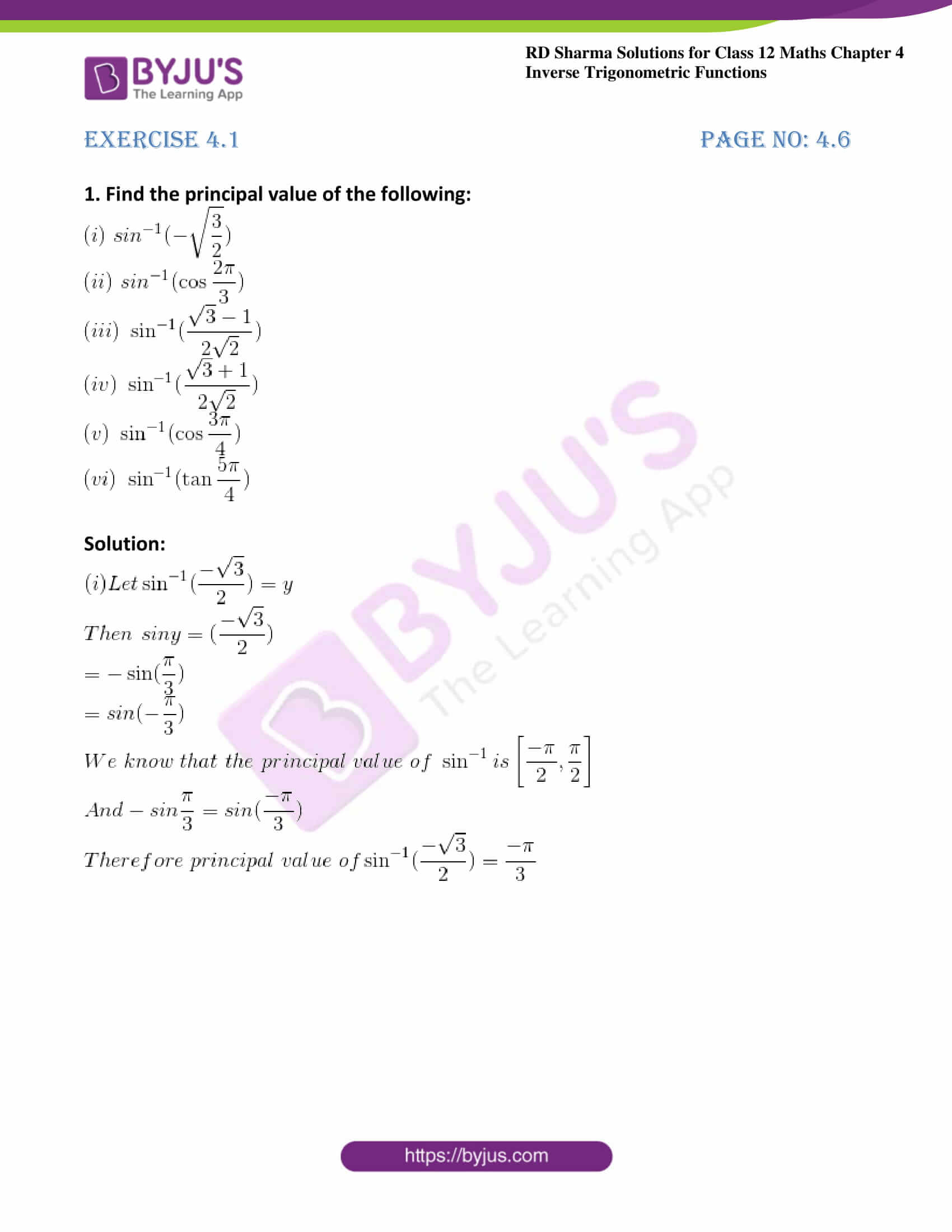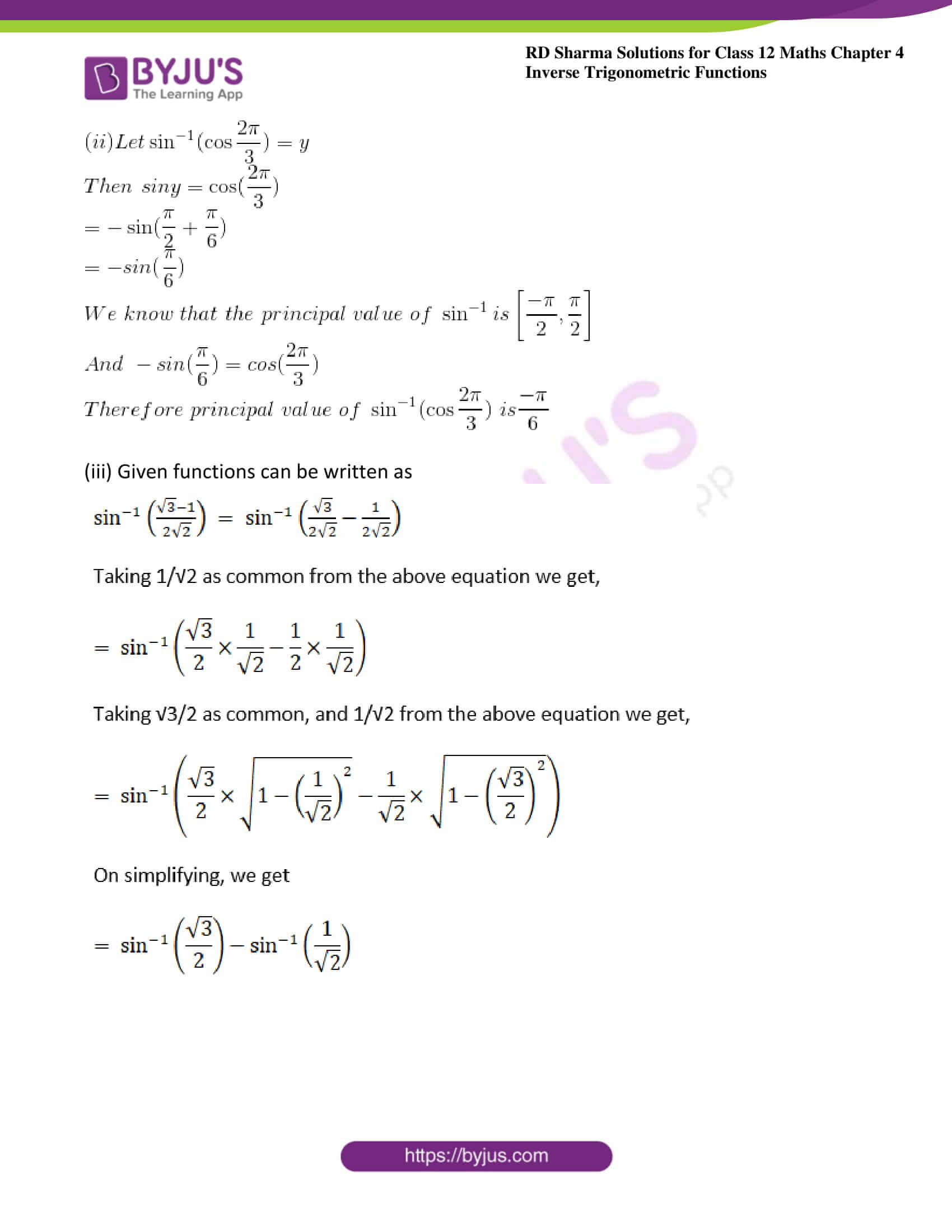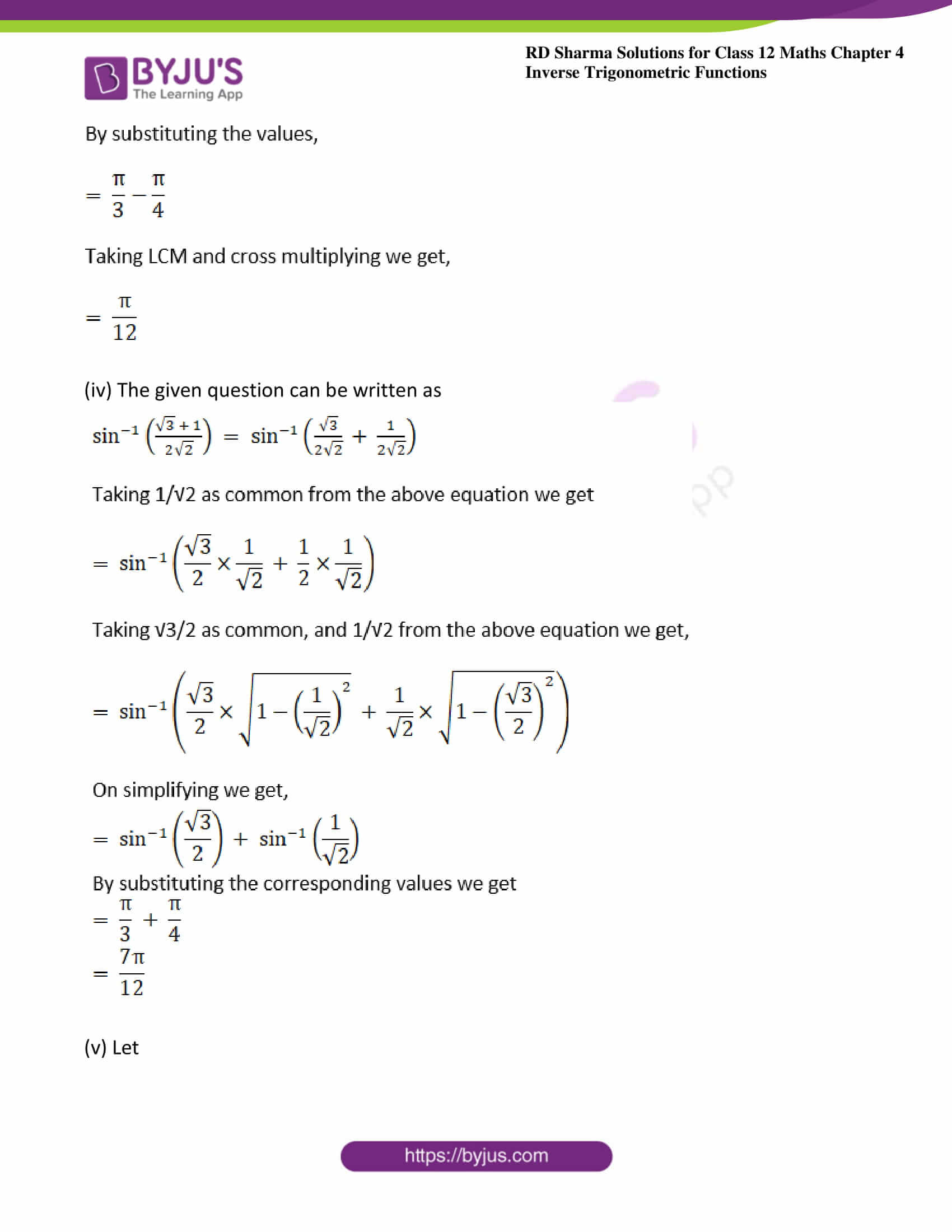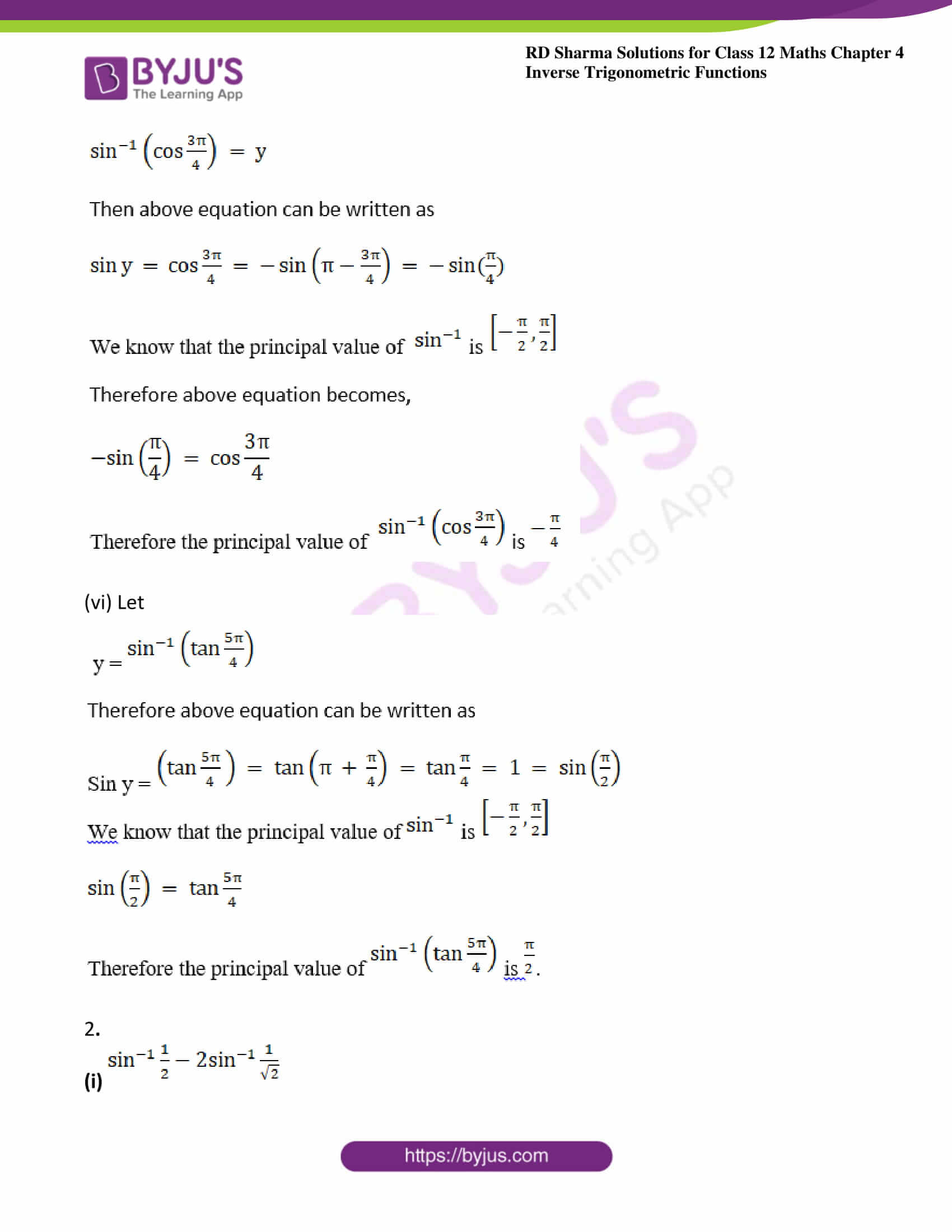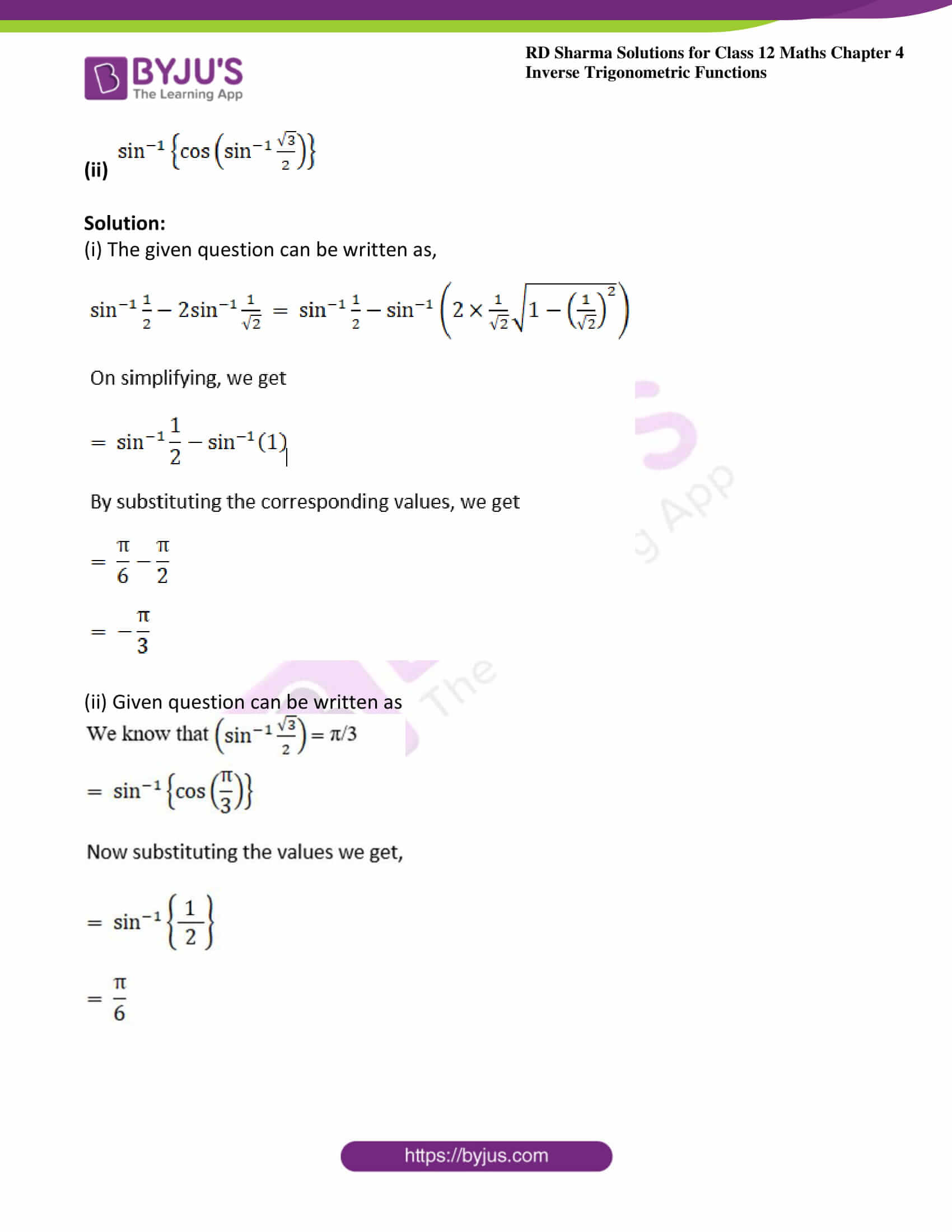### Access answers to Maths RD Sharma Solutions For Class 12 Chapter 4 – Inverse Trigonometric Functions Exercise 4.1

1. Find the principal value of the following: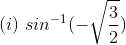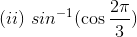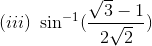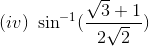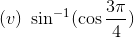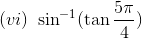Solution: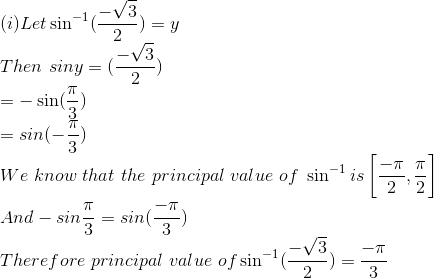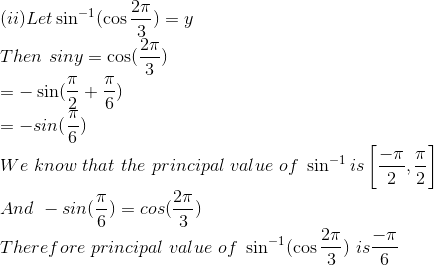(iii) Given functions can be written as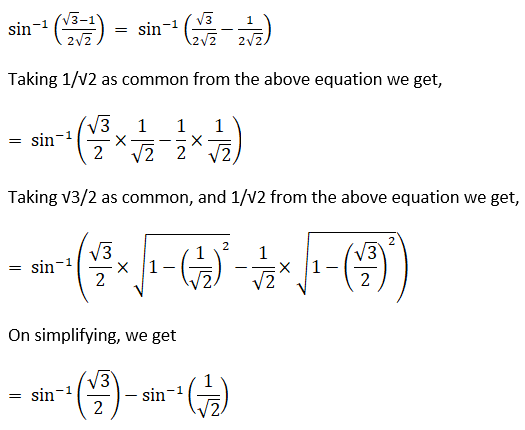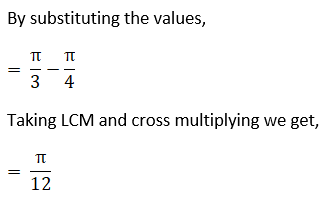(iv) The given question can be written as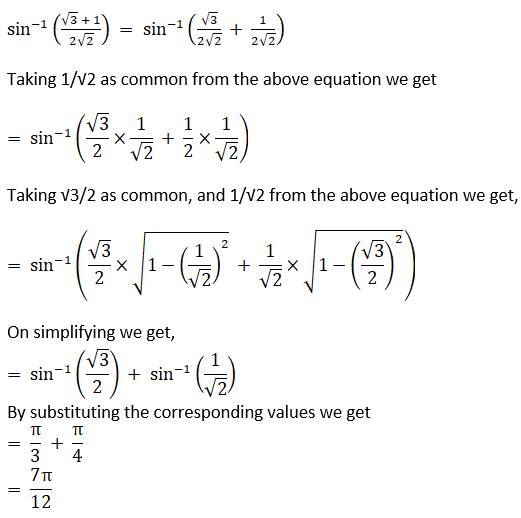(v) Let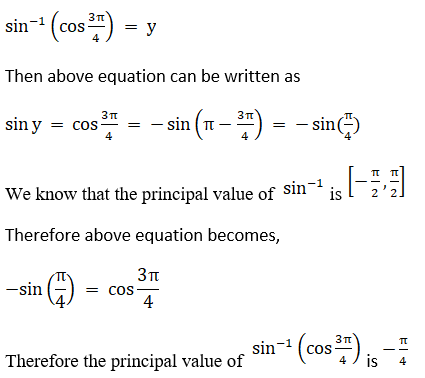(vi) Let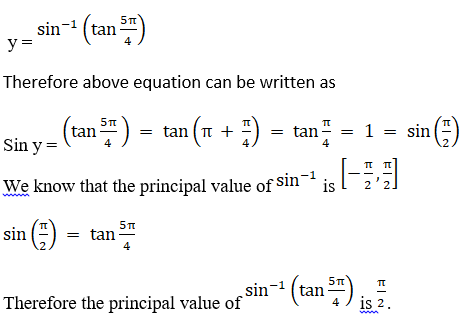2.

(i)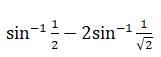(ii)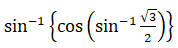Solution:

(i) The given question can be written as,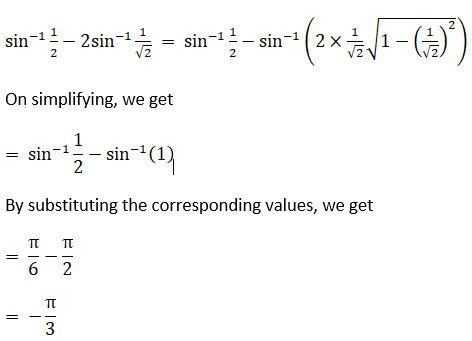(ii) Given question can be written as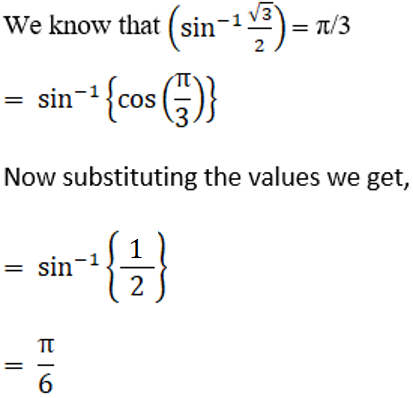### Access other exercises of RD Sharma Solutions For Class 12 Chapter 4 – Inverse Trigonometric Functions

Exercise 4.2 Solutions

Exercise 4.3 Solutions

Exercise 4.4 Solutions

Exercise 4.5 Solutions

Exercise 4.6 Solutions

Exercise 4.7 Solutions

Exercise 4.8 Solutions

Exercise 4.9 Solutions

Exercise 4.10 Solutions

Exercise 4.11 Solutions

Exercise 4.12 Solutions

Exercise 4.13 Solutions

Exercise 4.14 Solutions